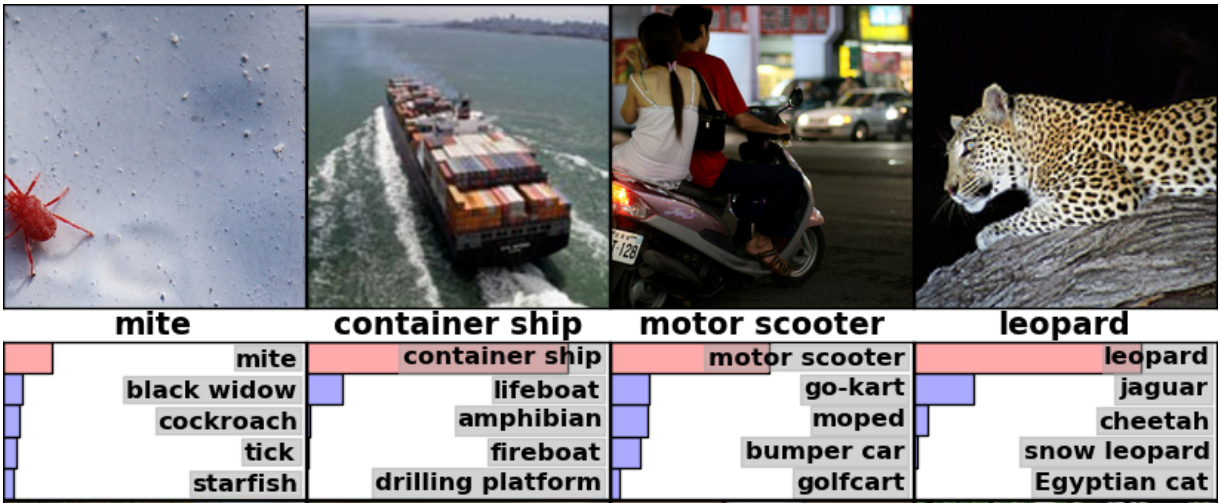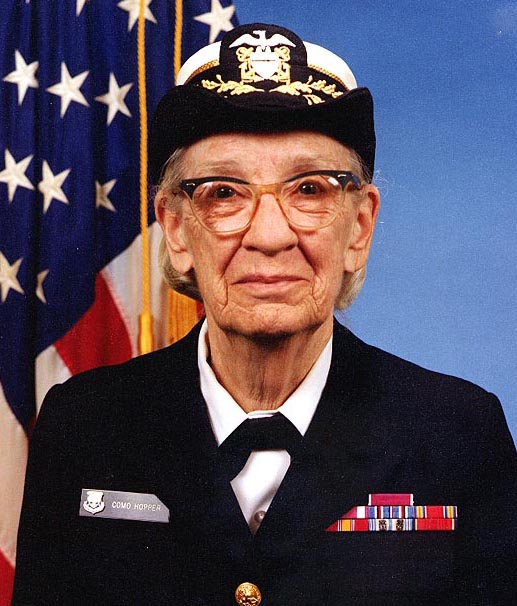Watch talks from the 2019 TensorFlow Dev Summit

# 图像识别

Inception-v3 使用 2012 年的数据针对 ImageNet 大型视觉识别挑战赛训练而成。它处理的是标准的计算机视觉任务，在此类任务中，模型会尝试将所有图像分成 1000 个类别，如“斑马”、“斑点狗”和“洗碗机”。例如，以下是 AlexNet 对某些图像进行分类的结果：## 使用 Python API

cd models/tutorials/image/imagenet
python classify_image.pygiant panda, panda, panda bear, coon bear, Ailuropoda melanoleuca (score = 0.88493)
indri, indris, Indri indri, Indri brevicaudatus (score = 0.00878)
lesser panda, red panda, panda, bear cat, cat bear, Ailurus fulgens (score = 0.00317)
custard apple (score = 0.00149)
earthstar (score = 0.00127)


## 使用 C++ API

curl -L "https://storage.googleapis.com/download.tensorflow.org/models/inception_v3_2016_08_28_frozen.pb.tar.gz" |
tar -C tensorflow/examples/label_image/data -xz


bazel build tensorflow/examples/label_image/...


bazel-bin/tensorflow/examples/label_image/label_image


I tensorflow/examples/label_image/main.cc:206] military uniform (653): 0.834306
I tensorflow/examples/label_image/main.cc:206] mortarboard (668): 0.0218692
I tensorflow/examples/label_image/main.cc:206] academic gown (401): 0.0103579
I tensorflow/examples/label_image/main.cc:206] pickelhaube (716): 0.00800814
I tensorflow/examples/label_image/main.cc:206] bulletproof vest (466): 0.00535088bazel-bin/tensorflow/examples/label_image/label_image --image=my_image.png


// Given an image file name, read in the data, try to decode it as an image,
// resize it to the requested size, and then scale the values as desired.
Status ReadTensorFromImageFile(string file_name, const int input_height,
const int input_width, const float input_mean,
const float input_std,
std::vector<Tensor>* out_tensors) {
tensorflow::GraphDefBuilder b;


  string input_name = "file_reader";
string output_name = "normalized";
b.opts().WithName(input_name));


  // Now try to figure out what kind of file it is and decode it.
const int wanted_channels = 3;
if (tensorflow::StringPiece(file_name).ends_with(".png")) {
} else {
// Assume if it's not a PNG then it must be a JPEG.
}
// Now cast the image data to float so we can do normal math on it.
tensorflow::Node* float_caster = tensorflow::ops::Cast(
// The convention for image ops in TensorFlow is that all images are expected
// to be in batches, so that they're four-dimensional arrays with indices of
// [batch, height, width, channel]. Because we only have a single image, we
// have to add a batch dimension of 1 to the start with ExpandDims().
tensorflow::Node* dims_expander = tensorflow::ops::ExpandDims(
float_caster, tensorflow::ops::Const(0, b.opts()), b.opts());
// Bilinearly resize the image to fit the required dimensions.
tensorflow::Node* resized = tensorflow::ops::ResizeBilinear(
dims_expander, tensorflow::ops::Const({input_height, input_width},
b.opts().WithName("size")),
b.opts());
// Subtract the mean and divide by the scale.
tensorflow::ops::Div(
tensorflow::ops::Sub(
resized, tensorflow::ops::Const({input_mean}, b.opts()), b.opts()),
tensorflow::ops::Const({input_std}, b.opts()),
b.opts().WithName(output_name));


  // This runs the GraphDef network definition that we've just constructed, and
// returns the results in the output tensor.
tensorflow::GraphDef graph;
TF_RETURN_IF_ERROR(b.ToGraphDef(&graph));


  std::unique_ptr<tensorflow::Session> session(
tensorflow::NewSession(tensorflow::SessionOptions()));
TF_RETURN_IF_ERROR(session->Create(graph));
TF_RETURN_IF_ERROR(session->Run({}, {output_name}, {}, out_tensors));
return Status::OK();


// Reads a model graph definition from disk, and creates a session object you
// can use to run it.
std::unique_ptr<tensorflow::Session>* session) {
tensorflow::GraphDef graph_def;
return tensorflow::errors::NotFound("Failed to load compute graph at '",
graph_file_name, "'");
}


  session->reset(tensorflow::NewSession(tensorflow::SessionOptions()));
Status session_create_status = (*session)->Create(graph_def);
if (!session_create_status.ok()) {
return session_create_status;
}
return Status::OK();
}


GetTopLabels() 函数很像图像加载，只是在本例中，我们想要获取运行主图得到的结果，并将其转换成得分最高的标签的排序列表。与图像加载器类似，该函数可创建一个 GraphDefBuilder，向其添加几个节点，然后运行较短的图，从而获取一对输出张量。在本例中，它们分别表示最高结果的经过排序的得分和索引位置。

// Analyzes the output of the Inception graph to retrieve the highest scores and
// their positions in the tensor, which correspond to categories.
Status GetTopLabels(const std::vector<Tensor>& outputs, int how_many_labels,
Tensor* indices, Tensor* scores) {
tensorflow::GraphDefBuilder b;
string output_name = "top_k";
tensorflow::ops::TopK(tensorflow::ops::Const(outputs, b.opts()),
how_many_labels, b.opts().WithName(output_name));
// This runs the GraphDef network definition that we've just constructed, and
// returns the results in the output tensors.
tensorflow::GraphDef graph;
TF_RETURN_IF_ERROR(b.ToGraphDef(&graph));
std::unique_ptr<tensorflow::Session> session(
tensorflow::NewSession(tensorflow::SessionOptions()));
TF_RETURN_IF_ERROR(session->Create(graph));
// The TopK node returns two outputs, the scores and their original indices,
// so we have to append :0 and :1 to specify them both.
std::vector<Tensor> out_tensors;
TF_RETURN_IF_ERROR(session->Run({}, {output_name + ":0", output_name + ":1"},
{}, &out_tensors));
*scores = out_tensors;
*indices = out_tensors;
return Status::OK();


PrintTopLabels() 函数会采用这些经过排序的结果，并以友好的方式输出这些结果。CheckTopLabel() 函数与其极为相似，但出于调试目的，需确保最有可能的标签是我们预期的值。

int main(int argc, char* argv[]) {
// We need to call this to set up global state for TensorFlow.
tensorflow::port::InitMain(argv, &argc, &argv);
Status s = tensorflow::ParseCommandLineFlags(&argc, argv);
if (!s.ok()) {
LOG(ERROR) << "Error parsing command line flags: " << s.ToString();
return -1;
}

// First we load and initialize the model.
std::unique_ptr<tensorflow::Session> session;
string graph_path = tensorflow::io::JoinPath(FLAGS_root_dir, FLAGS_graph);
return -1;
}


  // Get the image from disk as a float array of numbers, resized and normalized
// to the specifications the main graph expects.
std::vector<Tensor> resized_tensors;
string image_path = tensorflow::io::JoinPath(FLAGS_root_dir, FLAGS_image);
image_path, FLAGS_input_height, FLAGS_input_width, FLAGS_input_mean,
FLAGS_input_std, &resized_tensors);
return -1;
}
const Tensor& resized_tensor = resized_tensors;


  // Actually run the image through the model.
std::vector<Tensor> outputs;
Status run_status = session->Run({ {FLAGS_input_layer, resized_tensor}},
{FLAGS_output_layer}, {}, &outputs);
if (!run_status.ok()) {
LOG(ERROR) << "Running model failed: " << run_status;
return -1;
}


  // This is for automated testing to make sure we get the expected result with
// the default settings. We know that label 866 (military uniform) should be
// the top label for the Admiral Hopper image.
if (FLAGS_self_test) {
bool expected_matches;
Status check_status = CheckTopLabel(outputs, 866, &expected_matches);
if (!check_status.ok()) {
LOG(ERROR) << "Running check failed: " << check_status;
return -1;
}
if (!expected_matches) {
LOG(ERROR) << "Self-test failed!";
return -1;
}
}


  // Do something interesting with the results we've generated.
Status print_status = PrintTopLabels(outputs, FLAGS_labels);


  if (!print_status.ok()) {
LOG(ERROR) << "Running print failed: " << print_status;
return -1;
}